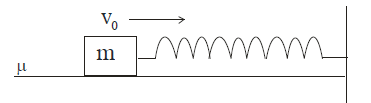# Work energy and power Problems

In this page we have Work energy and power Problems . Hope you like them and do not forget to like , social share and comment at the end of the page.
Question 1
The Potential Energy U in Joule of a particle of mass m=2 kg moving in the x-y plane is given by the formula
$U=6x + 8y+5$
Here x and y are coordinates of the particle in meter
The particle is at (4, 6) at t=0 and v0=0
i and j are the unit vectors across x and y axis respectively
(a) Find the force acting on the particle
(b) Find the kinetic energy of the particle at time t
(c) Find the time at which it crosses x axis and y axis and Kinetic energy at that points
(d) Find the coordinates of the particle as a function of time t
(e) Find the Potential Energy of the particle at time t=2 sec

Question 2
A body of mass m is displaced from Position A to B by the forces F1  and F2
Given
Position Vector of Point A=i+2j+3k
Position Vector of Point B=3i+j+2k
F1= i+2j+3k
F2= i+j-2k
Find the total work done by the resultant forces

Question 3
A block of mass m is placed on a inclined plane of angle α. The Friction Coefficient between the block and surface of the incline plane is μ. The block is placed at height H From the bottom of the incline plane. Find the velocity with which the block reaches the bottom of the incline

Question 4
A block moves horizontally on a rough floor. The Friction Coefficient between the block and surface is μ. The block strikes the light spring of spring constant K with velocity v(a) Find the maximum compression of the spring
(b) Find the work done by the Friction force
(c) Find the work done by the spring forces

Question 5
An object of mass M is given an initial velocity v0 on the rough horizontal surface in a positive x –axis direction. The Friction Coefficient between the object and surface is μ.
At t=0, x=0 and i is the unit vector across x axis
(a) Find the instantaneous Power P developed by the Friction forces
(b) Find the velocity of the object at time t
(c) Find the time taken by the object before coming to rest
(d) Find the total work done by the frictional forces
(e) Find the mean power developed by the frictional forces during the whole motion
(f) if μ varies as per the following
μ=x
Find the value of the x when instantaneous power is maximum

Question 6
A car of mass M accelerates starting from rest along +x axis .The engine is supplying constant Power W .Initially car is at x=0
(a) Find the position of the car as a function of time
(b) Find the velocity of car as a function of time
(c) Find the acceleration of the car as a function of time

Question 7
A uniform chain of mass M and length L is lying on a friction less horizontal table with one fourth of its length hanging over of edge of the table. Find the amount of work done to pull the hanging part of the chain up the table

Question 8
A box is dragged across a floor by a rope which makes an angle of 600 with the horizontal. The tension in the rope is 200 N while the box is dragged is 20 m.
(a) Find the work done
(b) What will be the work done if the rope is horizontal